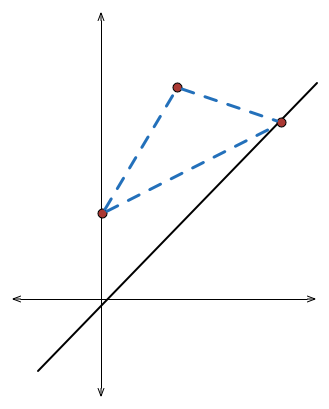# Mirror, Mirror

Geometry Level 5The minimum possible perimeter of a triangle with one vertex at $(3,9)$, one anywhere on the $y$-axis and one anywhere on the line $y = x$ is $a\sqrt{b}$, where $a$ and $b$ are positive coprime integers and $b$ is square free. Find $a + b.$

×